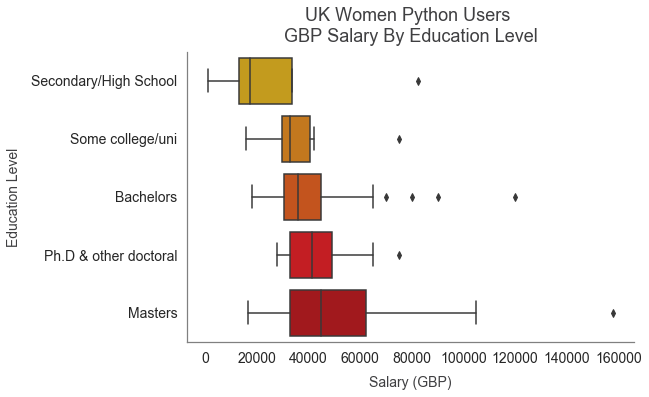# UK Python Users¶

## Data Source¶

This is an analysis of the 2019 StackOverflow survey data, available here.

The data includes information about StackOverflow users from across the world, including demographics, primary programming languages, salaries and more.

This analysis looks only at UK users who use Python and are employed full time.

The code to analyse the data and produce the charts is hidden by default but you can view the code by clicking on the Show Code button at the top left of the page.

In :
import numpy as np

import pandas as pd

# Show a chart created using matplotlib directly under the code that produces it
%matplotlib inline

# Import pyplot from the matplotlib library, for creating charts
from matplotlib import pyplot as plt

# Import seaborn for additional chart styles
import seaborn as sns; sns.set()

# Configure the aesthetics of the charts
plt.rcParams['figure.figsize'] = (18, 12)
plt.rcParams['figure.facecolor'] = '#FFFFFF'
plt.rcParams['figure.frameon'] = False
plt.rcParams['axes.facecolor'] = '#FFFFFF'
plt.rcParams['axes.spines.top'] = False
plt.rcParams['axes.spines.right'] = False
plt.rcParams['savefig.facecolor'] = '#FFFFFF'

sns.set(style="ticks", color_codes=True)
sns.set_context("notebook")

sns.set({ "figure.figsize": (12/1.5,8/1.5) })
sns.set_style("white", {'axes.edgecolor':'gray'})

# Read in the csv of the global data into a dataframe called s


## Overview¶

In :
# Create a new dataframe, p_uk, containing responses from UK users who use Python
# and are employed full time.
p_uk = s.loc[
(s['Country']=='United Kingdom') &
(s['LanguageWorkedWith'].str.contains('Python') &
(s['Employment']=='Employed full-time'))
]

In :
# How many UK Python users (employed full time) responded to the survey?
str(len(p_uk)) + ' UK Python users employed full time ('\
+  str(round(len(p_uk)/len(s)*100, 2))\
+ '% of all survey respondents) responded.'

Out:
'1772 UK Python users employed full time (1.99% of all survey respondents) responded.'

## Top 10 Tools¶

In :
# Separate each 'LanguageWorkedWith' value into a list of words,
# by splitting where there is a semi-colon
lang_lists = p_uk['LanguageWorkedWith'].str.split(";")

# Create an empty list called lang_col, that will be used to
# store one language per row in the subsequent 'for' loop
lang_col = []

# loop through each row, and each element in the list in each row,
# and add the language in each element to the lang_col list
for row in lang_lists:
for element in row:
lang_col.append(element)

# change the lang_col series into a dataframe
lang_col_df = pd.DataFrame(lang_col)

# Rename the column from '0' to 'Language'
lang_col_df.columns=['Language']

In :
# display a horizontal bar chart of the top 10 tools and their percentage
.plot.barh(color='#deaa02', alpha=0.8, width=0.9);

# adjust the style elements of the plot
plt.ylabel(None);
plt.xlabel('% UK Python Users', fontsize=14, color='#3F3F41');
plt.tick_params(labelsize=14, color='#3F3F41');
plt.legend().set_visible(False);
sns.despine(left=True);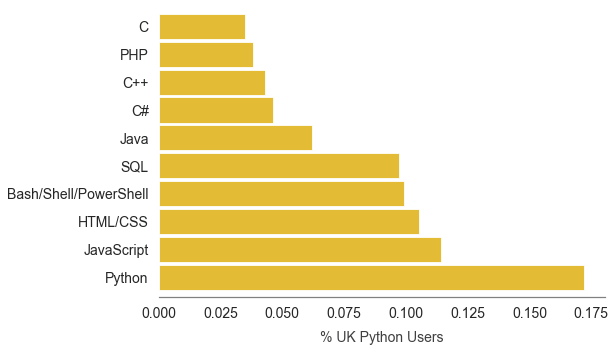## Top 10 Roles¶

In :
# How are UK Python users using code? Are they professional
# developers / use code as part of their work or something else?

# Create new dataframe of UK python users (who are employed full time) roles (DevTypes)
# where there is no missing value
devtypes_notmissing = pd.DataFrame(p_uk.loc[p_uk['DevType'].notnull(), 'DevType'])

# Separate each 'DevType' value into a list of words,
# by splitting where there is a semi-colon
devtype_lists = devtypes_notmissing['DevType'].str.split(";")

# Create an empty list called devtype_col, that will be used to
# store one role per row in the subsequent 'for' loop
devtype_col = []

# loop through each row, and each element in the list in each row,
# and add the role in each element to the devtype_col list
for row in devtype_lists:
for element in row:
devtype_col.append(element)

# change the devtype_col series into a dataframe
devtype_col_df = pd.DataFrame(devtype_col)

# Rename the column from '0' to 'Role'
devtype_col_df.columns=['Role']

In :
# display a horizontal bar chart of the top 10 roles and their percentage
.plot.barh(color='#de7b02', alpha=0.8, width=0.9);

# adjust the style elements of the plot
plt.ylabel(None);
plt.xlabel('% UK Python Users', fontsize=14, color='#3F3F41');
plt.tick_params(labelsize=14, color='#3F3F41');
plt.legend().set_visible(False);
sns.despine(left=True);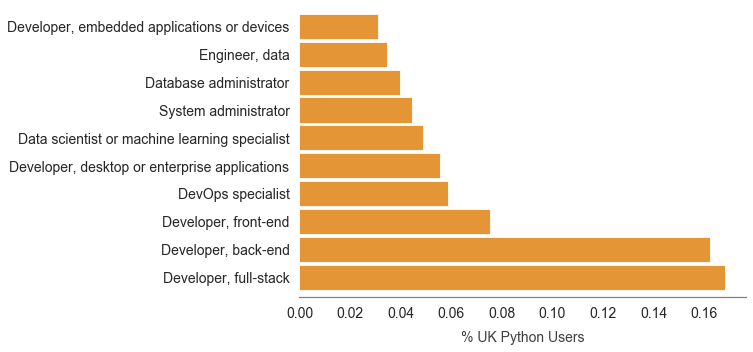## Gender¶

In :
# edit the gender values to shorter descriptions to make the plot more readable
p_uk.loc[p_uk.Gender ==\
'Woman;Man', 'Gender'] = 'Non-binary'
p_uk.loc[p_uk.Gender ==\
'Non-binary, genderqueer, or gender non-conforming', 'Gender'] = 'Non-binary'
p_uk.loc[p_uk.Gender ==\
'Woman;Non-binary, genderqueer, or gender non-conforming', 'Gender'] = 'Non-binary'
p_uk.loc[p_uk.Gender ==\
'Man;Non-binary, genderqueer, or gender non-conforming', 'Gender'] = 'Non-binary'

# display a horizontal bar chart of each gender and its percentage
p_uk['Gender'].value_counts(normalize=True).plot.barh(color='#de4b02', alpha=0.8, width=0.9);

# adjust the style elements of the plot
plt.ylabel(None);
plt.xlabel('% UK Python Users', fontsize=14, color='#3F3F41');
plt.tick_params(labelsize=14, color='#3F3F41');
plt.legend().set_visible(False);
sns.despine(left=True);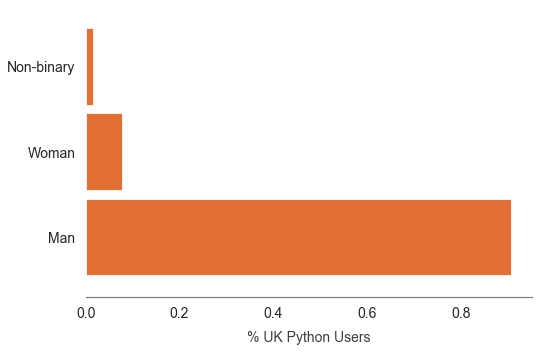## Age¶

In :
# display a density plot of the frequency of each age
p_uk['Age'].plot.kde(color='#de2702', alpha=0.8);

# adjust the style elements of the plot
plt.ylabel('Density', fontsize=14, color='#3F3F41');
plt.xlabel('Age', fontsize=14, color='#3F3F41');
plt.tick_params(labelsize=14, color='#3F3F41');
sns.despine();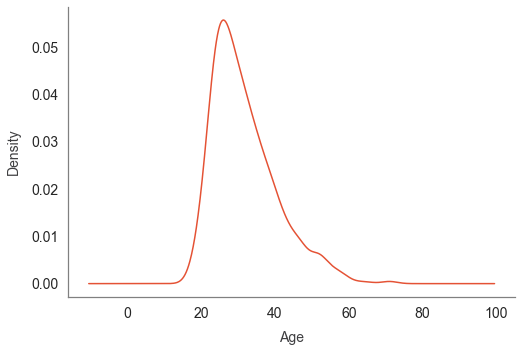## Education¶

In :
# disable the warning for the use of the chains below
pd.options.mode.chained_assignment = None  # default='warn'

# edit the education level values to shorter descriptions to make the plot more readable
p_uk.loc[
p_uk.EdLevel ==\
'Secondary school (e.g. American high school, German Realschule or Gymnasium, etc.)',\
'EdLevel'] = 'Secondary/High School'

p_uk.loc[
p_uk.EdLevel ==\
'Some college/university study without earning a degree',\
'EdLevel'] = 'Some college/uni'

p_uk.loc[
p_uk.EdLevel ==\
'Master’s degree (MA, MS, M.Eng., MBA, etc.)',\
'EdLevel'] = 'Masters'

p_uk.loc[p_uk.EdLevel == \
'Other doctoral degree (Ph.D, Ed.D., etc.)',\
'EdLevel'] = 'Ph.D & other doctoral'

p_uk.loc[p_uk.EdLevel == \
'I never completed any formal education',\
'EdLevel'] = 'No formal education completed'

p_uk.loc[p_uk.EdLevel ==\
"Bachelor’s degree (BA, BS, B.Eng., etc.)",\
'EdLevel'] = 'Bachelors'

p_uk['EdLevel'].value_counts(normalize=True)\
.plot.barh(color='#de0209', alpha=0.8, width=0.9);

# adjust the style elements of the plot
plt.ylabel(None);
plt.xlabel('% UK Python Users', fontsize=14, color='#3F3F41');
plt.tick_params(labelsize=14, color='#3F3F41');
plt.legend().set_visible(False);
sns.despine(left=True);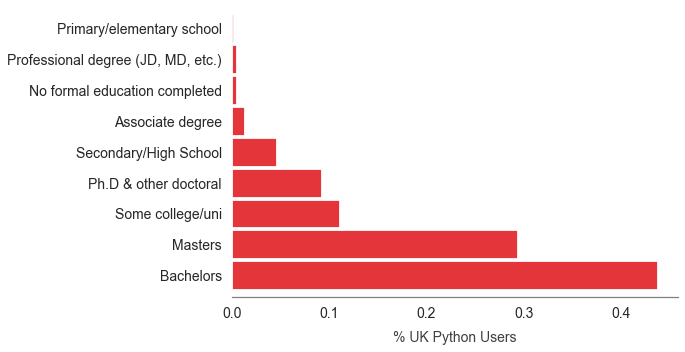## Salary¶

In :
# create a data frame of responses where the salary has been provided and
# the salary currency is GBP
p_uk_GBP_salaries = p_uk.loc[
(p_uk['CompTotal'].notnull()) &
(p_uk['CurrencySymbol']=='GBP')
]

# Display the median salary for those responses
'The median salary paid in GBP for UK Python users employed full time is '\
+ str(round(p_uk_GBP_salaries['CompTotal'].median()))

Out:
'The median salary paid in GBP for UK Python users employed full time is 45000'
In :
# display a boxplot of the non-null GBP salaries
sns.boxplot(p_uk_GBP_salaries['CompTotal'], color='#b80208');

# adjust the style elements of the plot
plt.xlabel('UK Python User Salary \n(Only those paid in GBP)',\
fontsize=14, color='#3F3F41');
plt.tick_params(labelsize=14, color='#3F3F41');
sns.despine(left=True);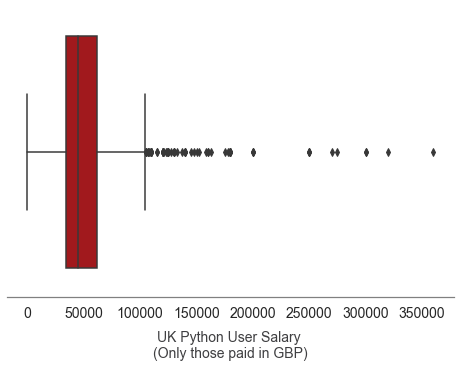Breaking that down by gender...

In :
# display a box plot of non-null GBP salaries per gender
with sns.color_palette(['#de0209', '#de4b02', '#deaa02']):
sns.boxplot(x='Gender', y='CompTotal', data=p_uk_GBP_salaries);

# adjust the style elements of the plot
plt.xlabel('Gender', fontsize=14, color='#3F3F41');
plt.ylabel('Salary', fontsize=14, color='#3F3F41');
plt.tick_params(labelsize=14, color='#3F3F41');
sns.despine();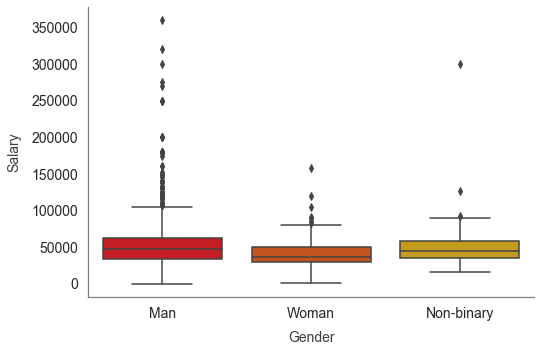Removing the outliers by only looking at salaries below £125k...

In :
# display a box plot of non-null GBP salaries per gender
# where the salary is under £125k, so that the distributions can
# be compared without the biggest outliers
with sns.color_palette(['#de0209', '#de4b02', '#deaa02']):
sns.boxplot(x='Gender',
y='CompTotal',
data=p_uk_GBP_salaries.loc[p_uk_GBP_salaries['CompTotal']<125000]
);

# adjust the style elements of the plot
plt.title('GBP Salary (<£125k) By Gender', fontsize=18, color='#3F3F41');
plt.xlabel('Gender', fontsize=14, color='#3F3F41');
plt.ylabel('Salary', fontsize=14, color='#3F3F41');
plt.tick_params(labelsize=14, color='#3F3F41');
sns.despine();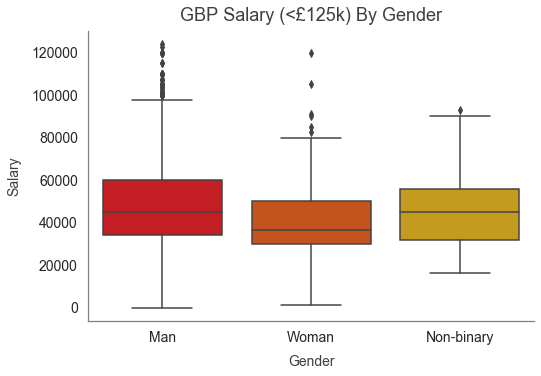Median salary per gender:

In :
# create a data frame of the median non-null GBP salary per gender
meds = pd.DataFrame(
p_uk_GBP_salaries.groupby(by='Gender')['CompTotal']\
.median()
)

# make the index (gender) a column
meds.reset_index(inplace=True)

meds.columns = ['Gender', 'Median Salary']

# display the data frame of median salaries per gender
meds

Out:
Gender Median Salary
0 Man 47000.0
1 Non-binary 45000.0
2 Woman 37000.0

Comparing the salary distributions of women by their education level...

In :
# create a dataframe containing only the non-null GBP salaries
# of UK python users who identify as a woman, and only
# keep the columns containing salary and education level
uk_python_women = p_uk_GBP_salaries.loc[
p_uk_GBP_salaries.Gender=='Woman',
['Gender', 'EdLevel', 'CompTotal']
]

# display a box plot of salary per education level
with sns.color_palette(['#deaa02', '#de7b02', '#de4b02', '#de0209', '#b80208']):
sns.boxplot(y='EdLevel',
x='CompTotal',
data=uk_python_women,
order=['Secondary/High School',
'Some college/uni',
'Bachelors',
'Ph.D & other doctoral',
'Masters']
);

# adjust the style elements of the plot
plt.title("UK Women Python Users \nGBP Salary By Education Level",
fontsize=18,
color='#3F3F41');
plt.ylabel('Education Level',
fontsize=14,
color='#3F3F41');
plt.xlabel('Salary (GBP)',
fontsize=14,
color='#3F3F41');
plt.tick_params(labelsize=14,
color='#3F3F41');
sns.despine();